Refraction through a Prism

# Our Objective:

(i) To study the angle of deviation (d) with angle of incidence (i) and to find the angle of minimum deviation (D) from i-d curve.

(ii) To find the refractive index of the material of the prism using A and D.

# The Theory:

## Prism

A prism is an optical element. It has polished flat surfaces that refract light. The traditional geometric shape of a prism has a triangular base and two rectangular sides. It is called triangular prism.

A prism can be made from materials like glass, plastic and fluorite. It can be used to split light into its components.

## How a Prism Works

When light travels from one medium to another medium, it is refracted and enters the new medium at a different angle. The degree of bending of the light's path depends on the angle that the incident beam of light makes with the surface of the prism, and on the ratio between the refractive indices of the two media. This is called Snell's law.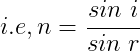where, n is the refractive index of the material of the prism.
i is the angle of incidence.
r is the angle of refraction.

The refractive index of many materials varies with the wavelength of the light used. This phenomenon is called dispersion. This causes light of different colors to be refracted differently and to leave the prism at different angles, creating an effect similar to a rainbow. This can be used to separate a beam of white light into its constituent spectrum of colors.

## The relation between Refractive Index (n), Angle of Prism (A) and Angle of Minimum Deviation (D)

Consider the following triangular prism.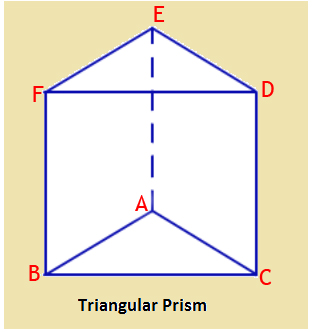The angle A between the two refracting surfaces ABFE and ACDE is called the angle of prism.

A ray of light suffers two refractions on passing through a prism. If KL be a monochromatic light falling on the side AB, it is refracted and travels along LM. It once again suffers refraction at M and emerges out along MN. The angle through which the emergent ray deviates from the direction of incident ray is called angle of deviation 'd'.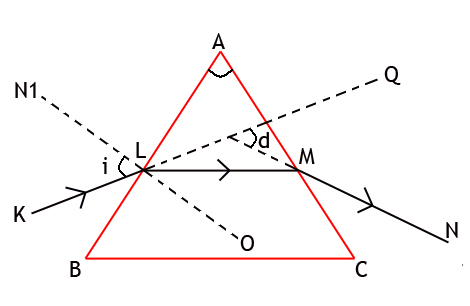As the angle of incidence is increased, angle of deviation 'd' decreases and reaches minimum value. If the angle of incidence is further increased, the angle of deviation is increased.

A graph is drawn between angle of incidence (i) and angle of deviation (d) by taking angle of incidence (i) along X-axis and angle of deviation (d) along Y-axis. It should be a curved graph.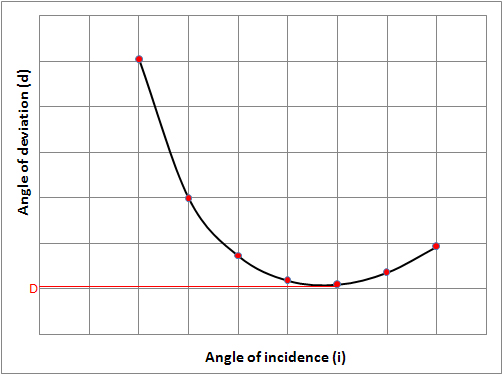The angle of minimum deviation is obtained from the graph. Let D be the angle of minimum deviation, then the refractive index (n) of the material of the prism is calculated using the formula,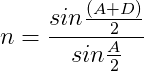# Learning outcomes:

• Students understand the working of a prism.
• Students will be better able to do the experiment in a real laboratory by understanding the procedure.